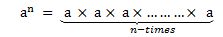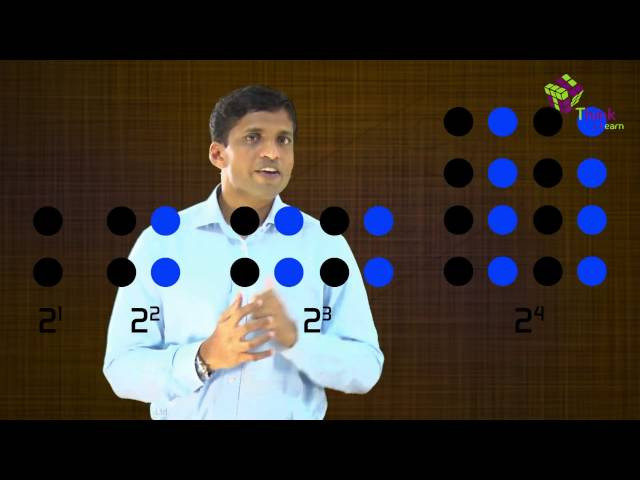# Exponents And Powers

Exponents are powers are the fundamentals of Maths. They are used to represent very large numbers or very small numbers in a simplified way.

Exponents are the repeated multiplication of any number. The number, 1,000,000 can be written as:

1,000,000 = 10 × 10 × 10 × 10 × 10 × 10 = 106, where 6 is the power of 10.

Thus, when 10 is multiplied 6 times we get 1,000,000. It can be expressed using power as 106. 10 is the base and 6 is the exponent. It is read as ’10 raised to the power of 6’. Hence, it is easy to express any integer as exponent with this method.

Exponents and Powers is an important topic for Class 7 and Class 8 students. Hence, they can learn the topic here in detail and clarify their doubts.

## Definition of Powers and Exponents

The exponent is a simple but powerful tool. It tells us how many times a number should be multiplied by itself to get the desired result. Thus any number ‘a’ raised to power ‘n’ can be expressed as:Here a is any number and n is a natural number.

an is also called the nth power of a.

‘a’ is the base and ‘n’ is the exponent or index or power.

‘a’ is multiplied ‘n’ times thereby exponentiation is the shorthand method of repeated multiplication.

## Laws of Exponents

The laws of exponents are demonstrated based on the powers they carry.

• Bases – multiplying the like ones – add the exponents and keep base same. (Multiplication Law)
• Bases – raise it with power to another – multiply the exponents and keep base same.
• Bases – dividing the like ones – ‘Numerator Exponent – Denominator Exponent’ and keep base same. (Division Law)

Let ‘a’ is any number or integer (positive or negative) and ‘m’,  ‘n’ are positive integers, denoting the power to the bases, then;

### Multiplication Law

As per the multiplication law of exponents, the product of two exponents with the same base and different powers equals to base raised to sum of the two powers or integers.

am × an  = am+n

### Division Law

When two exponents having same bases and different powers are divided, then it results in base raised to difference of the two powers.

am ÷ an  = am / an  = am-n

### Negative Exponent Law

Any base if has a negative power, then it results in reciprocal but with positive power or integer to the base.

a-m  = 1/am

## Exponents and Powers Rules

The rules of exponents are followed by the laws. Let us have a look on them with a brief explanation.

Suppose ‘a’ & ‘b’ are the integers and ‘m’ & ‘n’ are the values for powers, then the rules for exponents and powers are given by:

i) a0 = 1

As per this rule, if the power of any integer is zero, then the resulted output will be unity or one.

Example: 50 = 1

ii) (am)n = a(mn)

‘a’ raised to the power ‘m’ raised to the power ‘n’ is equal to ‘a’ raised to the power product of ‘m’ and ‘n’.

Example: (52)= 52 x 3

iii) am × bm =(ab)m

The product of ‘a’ raised to the power of ‘m’ and ‘b’ raised to the power ‘m’ is equal to the product of ‘a’ and ‘b’ whole raised to the power ‘m’.

Example: 52 × 62 =(5 x 6)2

iv) am/bm = (a/b)m

The division of ‘a’ raised to the power ‘m’ and ‘b’ raised to the power ‘m’ is equal to the division of ‘a’ by ‘b’ whole raised to the power ‘m’.

Example: 52/62 = (5/6)2

## Exponents and Power Solved Questions

Example 1: Write 7 x 7 x 7 x 7 x 7 x 7 x 7 x 7 in exponent form.

Solution:

In this problem 7s are written 8 times, so the problem can be rewritten as an exponent of 8.

7 x 7 x 7 x 7 x 7 x 7 x 7 x 7 = 78.

Example 2: Write below problems like exponents:

1. 3 x 3 x 3 x 3 x 3 x 3
2. 7 x 7 x 7 x 7 x 7
3. 10 x 10 x 10 x 10 x 10 x 10 x 10

Solution:

1. 3 x 3 x 3 x 3 x 3 x 3 = 36
2. 7 x 7 x 7 x 7 x 7 = 75
3. 10 x 10 x 10 x 10 x 10 x 10 x 10 = 107

Example 3: Simplify 253/53

Solution:

Using Law: am/bm = (a/b)m

253/53  can be written as (25/5)3  = 53 = 125.

## Exponents and Powers Applications

Scientific notation uses the power of ten expressed as exponents, so we need a little background before we can jump in. In this concept, we round out your knowledge of exponents, which we studied in previous classes.

The distance between the Sun and the Earth is 149,600,000 kilometers. The mass of the Sun is 1,989,000,000,000,000,000,000,000,000,000 kilograms. The age of the Earth is 4,550,000,000 years. These numbers are way too large or small to memorize in this way.  With the help of exponents and powers these huge numbers can be reduced to a very compact form and can be easily expressed in powers of 10.

Now, coming back to the examples we mentioned above, we can express the distance between the Sun and the Earth with the help of exponents and powers as following:

Distance between the Sun and the Earth 149,600,000 = 1.496× 10 × 10 × 10 × 10 × 10× 10 × 10 = 1.496× 108 kilometers.

Mass of the Sun: 1,989,000,000,000,000,000,000,000,000,000 kilograms = 1.989 × 1030 kilograms.

Age of the Earth:  4,550,000,000 years = 4. 55× 109 years

## Video LessonThis section covers the few important concepts related powers and exponents. For any further query on this topic install BYJU’S the learning app and excel your knowledge.

#### 1 Comment

1. Mohammed Al Maleki

Hi and thanks for this video